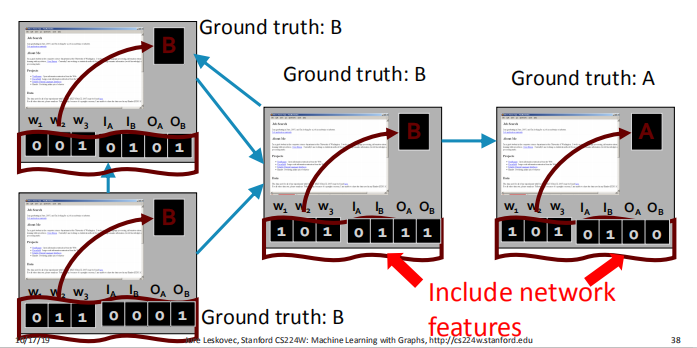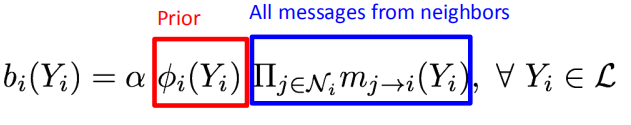# Stanford图机器学习公开课CS224W（六）笔记

## Lecture 6 -Message Passing and Node Classification

Posted by Yunlongs on December 1, 2020

# Message Passing and Node Classification

• Relational Classification
• Iterative classification
• Belief propagation

## 一.Collective Classification Overview

Markov假设： 节点$i$的标签$Y_i$依赖于它邻居节点$N_i$的标签

$P(Y_i||i) = P(Y_i||N_i)$

Collective classification涉及三个步骤：

1. Local Classifier: 分配初始标签
• 根据节点的特征进行标准分类，但不使用网络信息
2. Rleational Classifier：捕捉节点之间的关系
• 基于邻居节点的标签或特征学习出一个节点分类器，使用了网络信息
3. Collective Inference: 通过网络传递关系
• 迭代的对每个节点应用Relational classifier，最后网络结构将会影响到节点的预测结果

## 二.Relational Classifier

$P(Y_i=c) = \frac{1}{\sum_{(i,j)\in E} W(i,j)} \sum_{(i,j) \in E} W(i,j)P(Y_j = c)$
1. 对于有标签的节点，我们初始化为它们本身的标签$Y$。
2. 对于没有标签的节点，均匀地初始化$Y$
3. 以随机的顺序来更新所有的节点，直到收敛或者达到迭代的最大数

Challenge：

• 不保证收敛
• 此模型没有使用节点的特征信息

## 三.Iterative Classification

Iterative classification的主要思想： 基于节点$i$自身的特征和其邻居节点集合$N_i$的标签来进行分类

• 首先为每个节点创造一个特征向量$a_i$
• 然后使用特征向量$a_i$来训练一个分类器
• 对于节点的邻居，我们可以使用将它们的信息进行累积：count, mode, proportion, mean, exists…

### 3.1 具体流程

BootStrp 阶段：

• 为每个节点分配一个特征向量$a_i$
• 根据特征向量，使用分类器$f(a_i)$ (e.g.,SVM,KNN) 来为节点$i$计算最佳的标签$Y_i$

Iteration 阶段: 对于每个节点$i$，重复地更新节点特征向量$a_i$，然后根据$f(a_i)$来更新节点标签$Y_i$

### 3.2 Example:Web Page classification

1. 第一部分就为该节点中关键词的个数
2. 第二部分为一个表示邻居节点标签的向量$(I_A,I_B,O_A,O_B)$。其中$I=In,O=Out$，例如，$I_A=1$就代表着至少有一个指向此节点的邻居节点标记为$A$。## 四.Loopy Belief Propagation

### 4.1 Notation

Label-label potential matrix $\psi$: 来表征节点和其邻居节点之间的依赖关系。$\psi (Y_i,Y_j)$为在给定具有状态为$Y_i$的邻居节点$i$的情况下，节点$j$为状态$Y_j$的概率。

Prior belief $\phi$: 节点$i$为状态$Y_i$的概率$\phi_i(Y_i)$

$m_{i \rightarrow j}(Y_j)$ 为当$j$为状态$Y_j$时，节点$i$传递给$j$的消息

$\mathcal{L}$ 为所有状态的集合

### 4.2 算法流程Notice： 当图中存在回路的时候，Belief Propagation可能会出错，但是通常在实际使用的过程中，因为边之间的弱连接和长回路，造成的影响通常很弱。